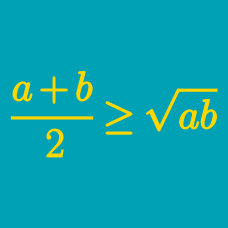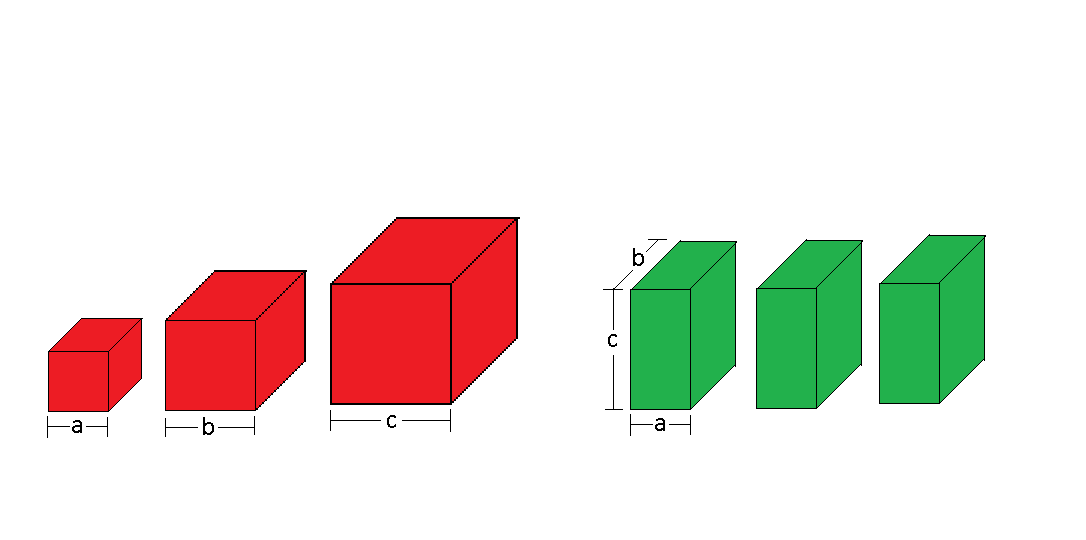Algebra

# Power Mean Inequalities: Level 3 ChallengesA jelly shop sells its products in two different sets: 3 red jelly cubes and 3 green jelly cuboids.

The 3 red cubes are of side lengths $a while the 3 green cuboids are identical with dimensions $a\times b\times c,$ as shown above.

Which option would give you more jelly?

Given that $a_1,a_2,a_3,a_4 > 0$, find the maximum constant $N$ for which the following inequality always holds true:

$\frac{a_1a_2(a_3^2+a_4^2)+a_3a_4(a_1^2+a_2^2)}{a_1a_2a_3a_4} \geq N.$

Let $a$ and $b$ be positive real numbers such that $a+b=1$. Find the maximum value of ${ a }^{ b }{ b }^{ a }+{ a }^{ a }{ b }^{ b }$.

$\large \dfrac{1}{a^2}+\dfrac{1}{b^2}+\dfrac{1}{c^2}+\dfrac{2(a^2+b^2+c^2)}{3}$ Let $a,b$ and $c$ be positive reals satisfy $a+b+c=3$. Find the minimum value of the expression above.

Give your answer to 2 decimal places

For some positive reals satisfying $\displaystyle{ \sum_{i=1}^{24} x_i=1}$, determine the maximum possible value of $\displaystyle{ \left (\sum_{i=1}^{24}\sqrt{x_i} \right ) \left (\sum_{i=1}^{24} \frac{1}{\sqrt{1+x_i}} \right )}.$

There exist 3 positive numbers such that their sum is 8 and their product is 27.

Is this true?

×

Problem Loading...

Note Loading...

Set Loading...